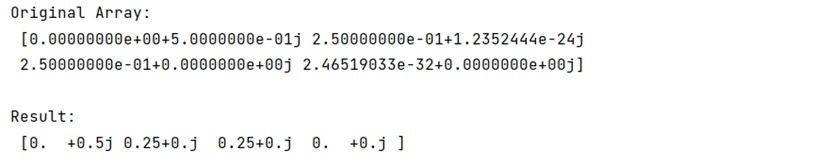# Set very low values to zero in NumPy

Given a NumPy array, we have to set very low values to zero in it.
Submitted by Pranit Sharma, on February 25, 2023

NumPy is an abbreviated form of Numerical Python. It is used for different types of scientific operations in python. Numpy is a vast library in python which is used for almost every kind of scientific or mathematical operation. It is itself an array which is a collection of various methods and functions for processing the arrays.

## Setting very low values to zero

Suppose that we are given a complex numpy array where elements are in the form of x + ij, we need to find a fast way to set the super low value to zero.

For this purpose, we need to define a limit for the imaginary part of the value, if the absolute values of the real and imaginary part of the values are less than the limit, we will set these values to zero.

Let us understand with the help of an example,

## Python code to set very low values to zero in NumPy

```# Import numpy
import numpy as np

# Creating a numpy array
arr = np.array([0 +  0.5j, 0.25 + 1.2352444e-24j, 0.25+ 0j, 2.46519033e-32 + 0j])

# Display original array
print("Original Array:\n",arr,"\n")

# Defining a limit
lim = 1e-16

# Set super low values to zero
arr.real[abs(arr.real) < lim] = 0.0
arr.imag[abs(arr.imag) < lim] = 0.0

# Display result
print("Result:\n",arr)
```

Output:Languages: » C » C++ » C++ STL » Java » Data Structure » C#.Net » Android » Kotlin » SQL
Web Technologies: » PHP » Python » JavaScript » CSS » Ajax » Node.js » Web programming/HTML
Solved programs: » C » C++ » DS » Java » C#
Aptitude que. & ans.: » C » C++ » Java » DBMS
Interview que. & ans.: » C » Embedded C » Java » SEO » HR
CS Subjects: » CS Basics » O.S. » Networks » DBMS » Embedded Systems » Cloud Computing
» Machine learning » CS Organizations » Linux » DOS
More: » Articles » Puzzles » News/Updates